Next: Bibliography Up: Survey on Independent Component Previous: Definition of Cumulants

# Nomenclature:

PCA: Principal Component Analysis
ICA: Independent Component Analysis
pdf: probability density function
Variables and constants:
i: General-purpose index, also: imaginary unit
m: Dimension of the observed data
n: Dimension of the transformed component vector
t: Time or iteration index
x and y: General-purpose scalar random variables
yi: Output of the i-th neuron in a neural network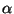: A scalar constant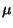: Learning rate constant or sequence
All the vectors are printed in boldface lowercase letters, and are column vectors: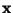: Observed data, an m-dimensional random vector
Also: the input vector of a neural network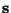: n-dimensional random vector of transformed components si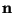: m-dimensional random noise vector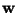: m-dimensional constant vector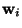: Weight vectors of a neural network indexed by i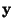: m-dimensional general-purpose random vector
Also: the output vector of a neural network
All the matrices are printed in boldface capital letters: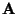: The constant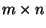mixing matrix in the ICA model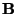: A transformed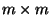mixing matrix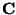: Covariance matrix of,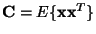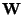: The weight matrix of an artificial neural network, with rows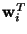Also: A general transformation matrix
Functions: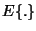: Mathematical expectation
f(.): A probability density function
fi(.): Marginal probability density functions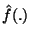: The characteristic function of a random variable
g(.): A scalar non-linear function
H(.): Differential entropy
I(.): Mutual information
J(.): Negentropy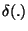: Kullback-Leibler divergence
JG(.): Generalized contrast function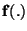: A general transformation from Rm to Rn
h(t): A FIR filter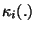: The i-th order cumulant of a scalar random variable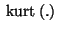: Kurtosis, or fourth-order cumulant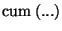: Cumulant (cross-cumulant) of several random variables
Other notation: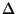: Change in parameter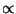: Proportional to (proportionality constant may change with t)
f': First derivative of function fNext: Bibliography Up: Survey on Independent Component Previous: Definition of Cumulants
Aapo Hyvarinen
1999-04-23